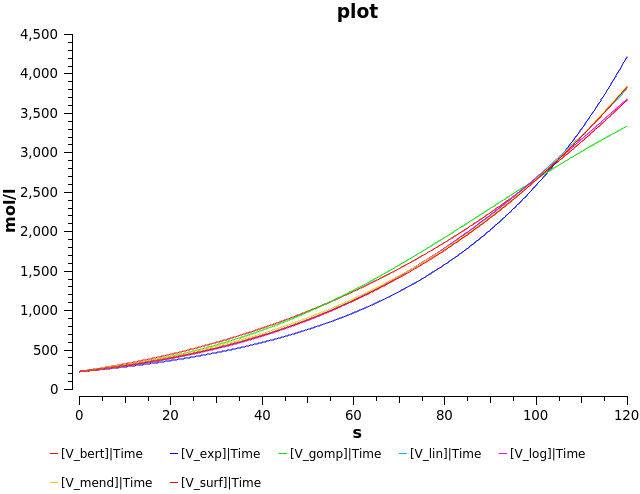## Murphy2016 - Differences in predictions of ODE models of tumor growthModel Identifier
BIOMD0000000671
Short description
Murphy2016 - Differences in predictions of ODE models of tumor growth
Comparison of 7 ODE models for tumour size. This models have been compared to experimental data.

This model is described in the article:

Murphy H, Jaafari H, Dobrovolny HM.
BMC Cancer 2016 Feb; 16: 163

Abstract:

While mathematical models are often used to predict progression of cancer and treatment outcomes, there is still uncertainty over how to best model tumor growth. Seven ordinary differential equation (ODE) models of tumor growth (exponential, Mendelsohn, logistic, linear, surface, Gompertz, and Bertalanffy) have been proposed, but there is no clear guidance on how to choose the most appropriate model for a particular cancer.We examined all seven of the previously proposed ODE models in the presence and absence of chemotherapy. We derived equations for the maximum tumor size, doubling time, and the minimum amount of chemotherapy needed to suppress the tumor and used a sample data set to compare how these quantities differ based on choice of growth model.We find that there is a 12-fold difference in predicting doubling times and a 6-fold difference in the predicted amount of chemotherapy needed for suppression depending on which growth model was used.Our results highlight the need for careful consideration of model assumptions when developing mathematical models for use in cancer treatment planning.

This model is hosted on BioModels Database and identified by: BIOMD0000000671.

To cite BioModels Database, please use: Chelliah V et al. BioModels: ten-year anniversary. Nucl. Acids Res. 2015, 43(Database issue):D542-8.

To the extent possible under law, all copyright and related or neighbouring rights to this encoded model have been dedicated to the public domain worldwide. Please refer to CC0 Public Domain Dedication for more information.

Format
SBML (L2V4)
Related Publication
• Differences in predictions of ODE models of tumor growth: a cautionary example.• Murphy H, Jaafari H, Dobrovolny HM
• BMC cancer , 2/ 2016 , Volume 16 , pages: 163 , PubMed ID: 26921070
• Department of Physics, Utica College, Utica, NY, USA. hemurphy@utica.edu.
• While mathematical models are often used to predict progression of cancer and treatment outcomes, there is still uncertainty over how to best model tumor growth. Seven ordinary differential equation (ODE) models of tumor growth (exponential, Mendelsohn, logistic, linear, surface, Gompertz, and Bertalanffy) have been proposed, but there is no clear guidance on how to choose the most appropriate model for a particular cancer.We examined all seven of the previously proposed ODE models in the presence and absence of chemotherapy. We derived equations for the maximum tumor size, doubling time, and the minimum amount of chemotherapy needed to suppress the tumor and used a sample data set to compare how these quantities differ based on choice of growth model.We find that there is a 12-fold difference in predicting doubling times and a 6-fold difference in the predicted amount of chemotherapy needed for suppression depending on which growth model was used.Our results highlight the need for careful consideration of model assumptions when developing mathematical models for use in cancer treatment planning.
Contributors
Submitter of the first revision: Emma Fairbanks
Submitter of this revision: Emma Fairbanks
Modellers: Emma Fairbanks

is (2 statements)
BioModels Database MODEL1708250001
BioModels Database BIOMD0000000671

isDescribedBy (2 statements)
PubMed 26921070
PubMed 26921070

hasTaxon (1 statement)
Taxonomy Homo sapiens

isPropertyOf (1 statement)
Experimental Factor Ontology neoplasm

hasProperty (1 statement)
Mathematical Modelling Ontology Ordinary differential equation model

Curation status
Curated

Modelling approach(es)

Tags

#### Connected external resources

SBGN view in Newt Editor

Name Description Size Actions

### Model files

BIOMD0000000671_url.xml SBML L2V4 representation of Murphy2016 - Differences in predictions of ODE models of tumor growth 27.68 KB Preview | Download

• Model originally submitted by : Emma Fairbanks
• Submitted: Aug 25, 2017 10:13:35 AM
##### Revisions

(*) You might be seeing discontinuous revisions as only public revisions are displayed here. Any private revisionsof this model will only be shown to the submitter and their collaborators.

Legends
: Variable used inside SBML models

Species
Species Initial Concentration/Amount
V log 220.0 mol
V surf 220.0 mol
V gomp 220.0 mol
V bert 220.0 mol
V lin 220.0 mol
V exp

Exponential Function
220.0 mol
V mend 220.0 mol
Reactions
Reactions Rate Parameters
V_log = a_log*V_log*(1-V_log/b_log) a_log*V_log*(1-V_log/b_log) b_log = 6920.0; a_log = 0.0295
V_surf = a_surf*V_surf/(V_surf+b_surf)^(1/3) a_surf*V_surf/(V_surf+b_surf)^(1/3) a_surf = 0.291; b_surf = 708.0
V_gomp = a_gomp*V_gomp*ln(b_gomp/(V_gomp+c_gomp)) a_gomp*V_gomp*ln(b_gomp/(V_gomp+c_gomp)) c_gomp = 10700.0; a_gomp = 0.0919; b_gomp = 15500.0
V_bert = a_bert*V_bert^(2/3)-b_bert*V_bert a_bert*V_bert^(2/3)-b_bert*V_bert a_bert = 0.2344; b_bert = 3.46E-19
V_lin = a_lin*V_lin/(V_lin+b_lin) a_lin*V_lin/(V_lin+b_lin) b_lin = 4300.0; a_lin = 132.0
V_exp = a_exp*V_exp a_exp*V_exp a_exp = 0.0246
V_mend = a_mend*V_mend^b_mend a_mend*V_mend^b_mend a_mend = 0.105; b_mend = 0.785Curator's comment:
(added: 07 Feb 2018, 15:13:23, updated: 07 Feb 2018, 15:13:23)
?Figure 3 of the reference publication has been reproduced. The simulations where performed and the plot was generated using Copasi 4.19 (Build 140).